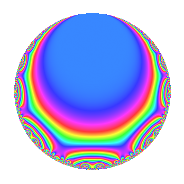Properties

 Label 8036.2.a.hLevel 8036 Weight 2 Character orbit 8036.a Self dual Yes Analytic conductor 64.168 Analytic rank 0 Dimension 3 CM No Inner twists 1

Related objects

Newspace parameters

 Level: $$N$$ = $$8036 = 2^{2} \cdot 7^{2} \cdot 41$$ Weight: $$k$$ = $$2$$ Character orbit: $$[\chi]$$ = 8036.a (trivial)

Newform invariants

 Self dual: Yes Analytic conductor: $$64.1677830643$$ Analytic rank: $$0$$ Dimension: $$3$$ Coefficient field: $$\Q(\zeta_{18})^+$$ Coefficient ring: $$\Z[a_1, a_2, a_3]$$ Coefficient ring index: $$1$$ Fricke sign: $$-1$$ Sato-Tate group: $\mathrm{SU}(2)$

$q$-expansion

Coefficients of the $$q$$-expansion are expressed in terms of a basis $$1,\beta_1,\beta_2$$ for the coefficient ring described below. We also show the integral $$q$$-expansion of the trace form.

 $$f(q)$$ $$=$$ $$q$$ $$+ ( 1 - \beta_{1} ) q^{3}$$ $$+ ( 2 \beta_{1} - 2 \beta_{2} ) q^{5}$$ $$+ ( -2 \beta_{1} + \beta_{2} ) q^{9}$$ $$+O(q^{10})$$ $$q$$ $$+ ( 1 - \beta_{1} ) q^{3}$$ $$+ ( 2 \beta_{1} - 2 \beta_{2} ) q^{5}$$ $$+ ( -2 \beta_{1} + \beta_{2} ) q^{9}$$ $$+ ( -2 + 2 \beta_{1} - 4 \beta_{2} ) q^{11}$$ $$+ ( 1 - \beta_{1} + 3 \beta_{2} ) q^{13}$$ $$+ ( -2 + 4 \beta_{1} - 4 \beta_{2} ) q^{15}$$ $$+ ( -1 - \beta_{2} ) q^{17}$$ $$+ ( 3 - 2 \beta_{1} - \beta_{2} ) q^{19}$$ $$+ ( 1 - \beta_{1} - 2 \beta_{2} ) q^{23}$$ $$+ ( 3 - 4 \beta_{1} ) q^{25}$$ $$+ 3 \beta_{2} q^{27}$$ $$+ ( -4 + 2 \beta_{1} ) q^{29}$$ $$+ ( 4 + 4 \beta_{1} - 4 \beta_{2} ) q^{31}$$ $$+ ( -2 + 8 \beta_{1} - 6 \beta_{2} ) q^{33}$$ $$+ ( -7 - 3 \beta_{1} + \beta_{2} ) q^{37}$$ $$+ ( -5 \beta_{1} + 4 \beta_{2} ) q^{39}$$ $$+ q^{41}$$ $$+ ( -1 + 4 \beta_{1} - \beta_{2} ) q^{43}$$ $$+ ( -6 + 4 \beta_{1} - 2 \beta_{2} ) q^{45}$$ $$+ ( 1 - 5 \beta_{1} - \beta_{2} ) q^{47}$$ $$+ ( 2 \beta_{1} - \beta_{2} ) q^{51}$$ $$+ ( 6 + 2 \beta_{1} + 2 \beta_{2} ) q^{53}$$ $$+ ( 12 - 8 \beta_{1} ) q^{55}$$ $$+ ( 8 - 4 \beta_{1} + \beta_{2} ) q^{57}$$ $$+ ( 2 + 4 \beta_{1} - 4 \beta_{2} ) q^{59}$$ $$+ ( 10 - 2 \beta_{1} ) q^{61}$$ $$+ ( -8 + 4 \beta_{1} + 2 \beta_{2} ) q^{65}$$ $$+ ( -6 - 4 \beta_{1} + 4 \beta_{2} ) q^{67}$$ $$+ ( 5 - \beta_{2} ) q^{69}$$ $$+ ( 4 + 2 \beta_{1} - 6 \beta_{2} ) q^{71}$$ $$+ ( 4 - 4 \beta_{1} + 4 \beta_{2} ) q^{73}$$ $$+ ( 11 - 7 \beta_{1} + 4 \beta_{2} ) q^{75}$$ $$+ ( 4 - 6 \beta_{1} + 6 \beta_{2} ) q^{79}$$ $$+ ( -3 + 3 \beta_{1} ) q^{81}$$ $$+ ( 6 - 4 \beta_{1} ) q^{83}$$ $$+ ( 2 - 2 \beta_{1} ) q^{85}$$ $$+ ( -8 + 6 \beta_{1} - 2 \beta_{2} ) q^{87}$$ $$+ ( 1 - 5 \beta_{1} + 7 \beta_{2} ) q^{89}$$ $$+ ( 4 \beta_{1} - 8 \beta_{2} ) q^{93}$$ $$+ ( -2 + 10 \beta_{1} - 12 \beta_{2} ) q^{95}$$ $$+ ( -1 - 5 \beta_{1} + 4 \beta_{2} ) q^{97}$$ $$+ ( -6 + 10 \beta_{1} - 2 \beta_{2} ) q^{99}$$ $$+O(q^{100})$$ $$\operatorname{Tr}(f)(q)$$ $$=$$ $$3q$$ $$\mathstrut +\mathstrut 3q^{3}$$ $$\mathstrut +\mathstrut O(q^{10})$$ $$3q$$ $$\mathstrut +\mathstrut 3q^{3}$$ $$\mathstrut -\mathstrut 6q^{11}$$ $$\mathstrut +\mathstrut 3q^{13}$$ $$\mathstrut -\mathstrut 6q^{15}$$ $$\mathstrut -\mathstrut 3q^{17}$$ $$\mathstrut +\mathstrut 9q^{19}$$ $$\mathstrut +\mathstrut 3q^{23}$$ $$\mathstrut +\mathstrut 9q^{25}$$ $$\mathstrut -\mathstrut 12q^{29}$$ $$\mathstrut +\mathstrut 12q^{31}$$ $$\mathstrut -\mathstrut 6q^{33}$$ $$\mathstrut -\mathstrut 21q^{37}$$ $$\mathstrut +\mathstrut 3q^{41}$$ $$\mathstrut -\mathstrut 3q^{43}$$ $$\mathstrut -\mathstrut 18q^{45}$$ $$\mathstrut +\mathstrut 3q^{47}$$ $$\mathstrut +\mathstrut 18q^{53}$$ $$\mathstrut +\mathstrut 36q^{55}$$ $$\mathstrut +\mathstrut 24q^{57}$$ $$\mathstrut +\mathstrut 6q^{59}$$ $$\mathstrut +\mathstrut 30q^{61}$$ $$\mathstrut -\mathstrut 24q^{65}$$ $$\mathstrut -\mathstrut 18q^{67}$$ $$\mathstrut +\mathstrut 15q^{69}$$ $$\mathstrut +\mathstrut 12q^{71}$$ $$\mathstrut +\mathstrut 12q^{73}$$ $$\mathstrut +\mathstrut 33q^{75}$$ $$\mathstrut +\mathstrut 12q^{79}$$ $$\mathstrut -\mathstrut 9q^{81}$$ $$\mathstrut +\mathstrut 18q^{83}$$ $$\mathstrut +\mathstrut 6q^{85}$$ $$\mathstrut -\mathstrut 24q^{87}$$ $$\mathstrut +\mathstrut 3q^{89}$$ $$\mathstrut -\mathstrut 6q^{95}$$ $$\mathstrut -\mathstrut 3q^{97}$$ $$\mathstrut -\mathstrut 18q^{99}$$ $$\mathstrut +\mathstrut O(q^{100})$$

Basis of coefficient ring in terms of $$\nu = \zeta_{18} + \zeta_{18}^{-1}$$:

 $$\beta_{0}$$ $$=$$ $$1$$ $$\beta_{1}$$ $$=$$ $$\nu$$ $$\beta_{2}$$ $$=$$ $$\nu^{2} - 2$$
 $$1$$ $$=$$ $$\beta_0$$ $$\nu$$ $$=$$ $$\beta_{1}$$ $$\nu^{2}$$ $$=$$ $$\beta_{2}\mathstrut +\mathstrut$$ $$2$$

Embeddings

For each embedding $$\iota_m$$ of the coefficient field, the values $$\iota_m(a_n)$$ are shown below.

For more information on an embedded modular form you can click on its label.

Label $$\iota_m(\nu)$$ $$a_{2}$$ $$a_{3}$$ $$a_{4}$$ $$a_{5}$$ $$a_{6}$$ $$a_{7}$$ $$a_{8}$$ $$a_{9}$$ $$a_{10}$$
1.1
 1.87939 −0.347296 −1.53209
0 −0.879385 0 0.694593 0 0 0 −2.22668 0
1.2 0 1.34730 0 3.06418 0 0 0 −1.18479 0
1.3 0 2.53209 0 −3.75877 0 0 0 3.41147 0
 $$n$$: e.g. 2-40 or 990-1000 Significant digits: Format: Complex embeddings Normalized embeddings Satake parameters Satake angles

Inner twists

This newform does not admit any (nontrivial) inner twists.

Atkin-Lehner signs

$$p$$ Sign
$$2$$ $$-1$$
$$7$$ $$-1$$
$$41$$ $$-1$$

Hecke kernels

This newform can be constructed as the intersection of the kernels of the following linear operators acting on $$S_{2}^{\mathrm{new}}(\Gamma_0(8036))$$:

 $$T_{3}^{3}$$ $$\mathstrut -\mathstrut 3 T_{3}^{2}$$ $$\mathstrut +\mathstrut 3$$ $$T_{5}^{3}$$ $$\mathstrut -\mathstrut 12 T_{5}$$ $$\mathstrut +\mathstrut 8$$ $$T_{11}^{3}$$ $$\mathstrut +\mathstrut 6 T_{11}^{2}$$ $$\mathstrut -\mathstrut 24 T_{11}$$ $$\mathstrut -\mathstrut 136$$